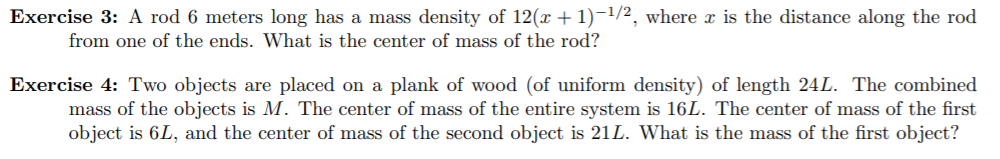# Exercise 3: A rod 6 meters long has a mass density of 12(x + 1)-1/2, where x is the distance along the rod from one of the ends. What is the center of mass of the rod? Exercise 4: Two objects are placed on a plank of wood (of uniform density) of length 24L. The combined mass of the objects is M. The center of mass of the entire system is 16L. The center of mass of the first object is 6L, and the center of mass of the second object is 21L. What is the mass of the first object?

Question

question 4help_outlineImage TranscriptioncloseExercise 3: A rod 6 meters long has a mass density of 12(x + 1)-1/2, where x is the distance along the rod from one of the ends. What is the center of mass of the rod? Exercise 4: Two objects are placed on a plank of wood (of uniform density) of length 24L. The combined mass of the objects is M. The center of mass of the entire system is 16L. The center of mass of the first object is 6L, and the center of mass of the second object is 21L. What is the mass of the first object? fullscreen

### Want to see this answer and more?

Experts are waiting 24/7 to provide step-by-step solutions in as fast as 30 minutes!*

*Response times vary by subject and question complexity. Median response time is 34 minutes and may be longer for new subjects.
Tagged in
MathCalculus

### Other